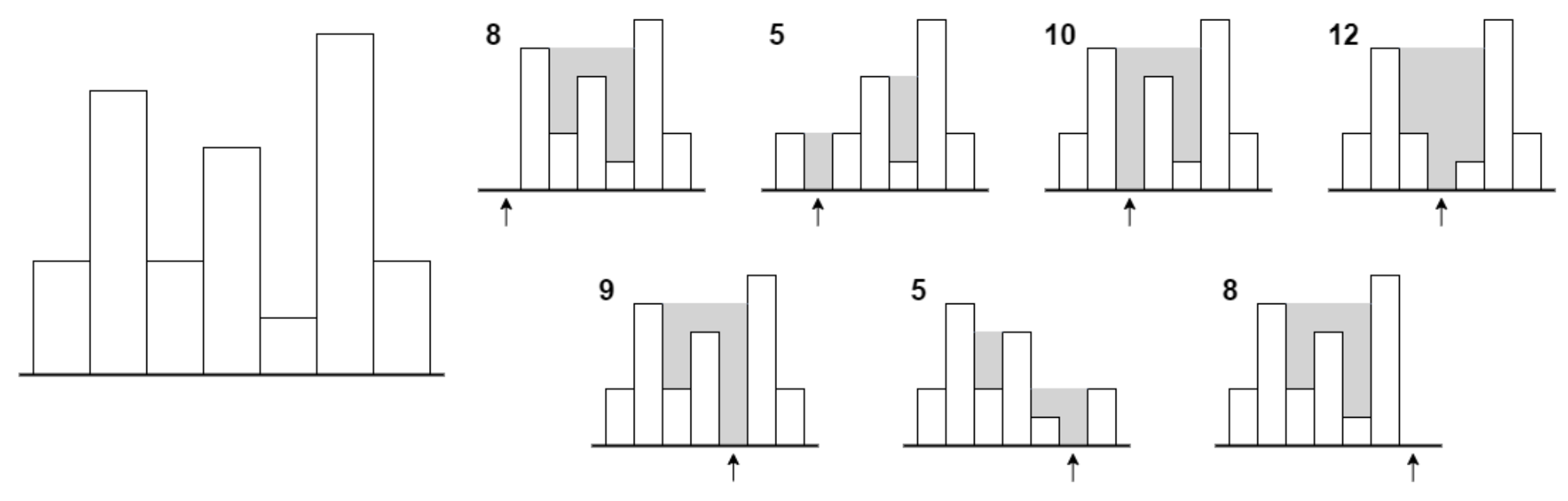시간 제한 메모리 제한 제출 정답 맞은 사람 정답 비율
3 초 512 MB 0 0 0 0.000%

## 문제

There are $n$ columns of width $1$ touching by sides arranged from left to right. Column that is $i$-th from the left has height $h_i$.

When the rain falls, water can get stuck in some places. It happens when there are short columns between two high columns.

Formally, water will remain in every point which is neither an interior nor boundary of a column, but for which there exist points on the same height belonging to some columns both on the left and on the right.

We are interested in an area of stuck water. If heights $h_i$ are all integers, this area is an integer as well. Superstition of elderly foretells that happiness is brought when the area of stuck water is divisible by $2$.

It seems it will be raining today. However, before that you plan to remove $k$ columns, i.e. change their heights to $0$. How many out of $n \choose k$ possibilities of removing columns will result in area of stuck water being even? Print the result modulo $10^9+7$.

## 입력

First line of input contains two integers $n$ and $k$ ($1 \leq n \leq 25\,000$, $0 \leq k \leq \min(25, n - 1)$), denoting number of columns and number of columns to be removed.

Second line of input contains $n$ integers $h_1, \ldots, h_n$ ($1 \leq h_i \leq 10^9$) --- heights of columns from left to right.

## 출력

You need to print one number --- number of possibilities modulo $10^9+7$ to remove $k$ columns so that area of stuck water will be even.

## 예제 입력 1

7 1
2 5 2 4 1 6 2


## 예제 출력 1

4


## 예제 입력 2

5 0
1 3 1 3 1


## 예제 출력 2

1


## 힌트

Explanation of first sample test:

Big picture on the left depicts initial setting of columns. Smaller pictures depict each of $7$ possibilities of removing one column (pointed by an arrow). Grey areas depict stuck water.

In $4$ cases area of stuck water (number next to each picture) is even.